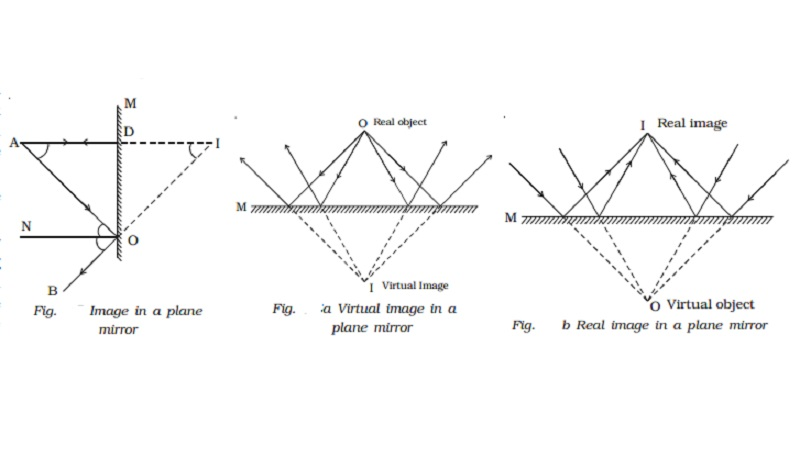Home | | Physics | | Physics | Image in a plane mirror

# Image in a plane mirrorLet us consider a point object A placed in front of a plane mirror M as shown in the Fig.. Consider a ray of light AO from the point object incident on the mirror and reflected along OB. Draw the normal ON to the mirror at O.

Image in a plane mirror

Let us consider a point object A placed in front of a plane mirror M as shown in the Fig.. Consider a ray of light AO from the point object incident on the mirror and reflected along OB. Draw the normal ON to the mirror at O.

The angle of incidence AON = angle of reflection BON

Another ray AD incident normally on the mirror at D is reflected back along DA. When BO and AD are produced backwards, they meet at I. Thus the rays reflected from M appear to come from a point I behind the mirror.From the figure

Angle AON = Angle  DAO  , alternate angles and Angle BON = Angle DIO= , corresponding angles it follows that Angle DAO = Angle DIO.

The triangles ODA and ODI are congruent

For a given position of the object, A and D are fixed points. Since AD = ID, the point I is also fixed. It should be noted that AO = OI. So the object and its image in a plane mirror are at equal perpendicular distances from the mirror..

Virtual and real images

An object placed in front of a plane mirror has an image behind the mirror. The rays reflected from the mirror do not actually meet through I, but only appear to meet and the image cannot be received on the screen, because the image is behind the mirror. This type of image is called an unreal or virtual image (Fig. a).If a convergent beam is incident on a plane mirror, the reflected rays pass through a point I in front of M, as shown in the Fig. b. In the Fig. a, a real object (divergent beam) gives rise to a virtual image. In the Fig. b, a virtual object (convergent beam) gives a real

image. Hence plane mirrors not only produce virtual images for real objects but also produce real images for virtual objects.

Characteristics of the image formed by a plane mirror

(i) Image formed by a plane mirror is as far behind the mirror as the object is in front of it and it is always virtual.

(ii) The image produced is laterally inverted.

(iii)                The minimum size of the mirror required to see the complete image of the object is half the size of the object.

(iv)If the mirror turns by an angle θ, the reflected ray turns through an angle 2θ.

(v)                  If an object is placed between two plane mirrors inclined at an  angle θ, then the number of images formed is n = 3600/ θ ? 1

Study Material, Lecturing Notes, Assignment, Reference, Wiki description explanation, brief detail

Related Topics